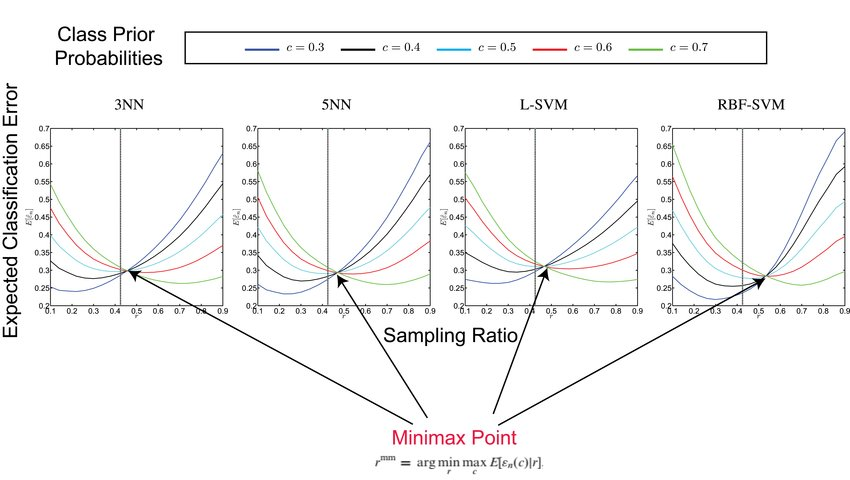# Separate sampling: its effects and one potential solution

Stratified Sampling

In classification studies it is typically a tacit assumption that sampling is random; indeed, it is commonplace for this assumption to be made throughout a text on classification. For instance, Devroye et al. declare on page 2 of their text that all sampling is random (Devroye, 1996). The assumption is so pervasive that it can be applied without mention. With regard to the problem at hand, Duda et al. (2001) state, ‘In typical supervised pattern classification problems, the estimation of the prior probabilities presents no serious difficulties.’ But, in fact, there are often serious difficulties.

Under the assumption of random sampling, the data set, Sn = {(X1Y1), … , (XnYn)}, is drawn independently from a fixed distribution of feature-label pairs, (XY); in particular, this means that if a sample of size n is drawn for a binary classification problem, then the numbers of sample points, n0 and n1, in classes 0 and 1, respectively, are random variables such that n0 + n1 = n.  Thus, if the sample is large, we can expect the sampling ratio to be close to the prior probability.

Suppose the sampling is not random, in the sense that the ratios are chosen prior to sampling. In this ‘separate (stratified) sampling’ case, where the sample points are selected randomly from the two classes but, given the total sample size, the individual class counts n0 and n1 are not random. Then, in effect, we have no sensible estimate of class prior probability.

Why Is This Important?

Since our aim is to use the data to train a classifier, does the inability to consistently estimate c matter? Why is all of this a major issue for data science? It has been shown that the separate sampling with no incorporation of true class prior probabilities have a significant degradation in classification performance (here).

Unfortunately, separate sampling is ubiquitous in many fields including bioinformatics, in particular, with genomic classification, where a standard approach is to take samples from two classes, say, different types of cancer or different stages of cancer, for which the number of specimens in each class is not chosen randomly, and then to design a classifier. But, when the true class ratios are unknown, which is practically often the case, what can we do to mitigate the problem?

One Robust Solution

Let us define a sampling ratio, r, controlling the ratio between the number of samples in each class. Obviously, there will be different sensitivsities of different classification rules to the sampling ratio, r. Now, going beyond the case where the true class prevalences (i.e. class prior probabilities) are known or approximately known, consider the third implication, where one has no good idea concerning the class ratiosOne can see that there exists a sampling ratio (at least for the tested classification rules here) where class-conditional errors cross each other, and that's what is shown to be the "minimax" ratio. Of course, its suitability depends upon the classification rule and feature-label distribution.

Conclusion

Finally, given the ubiquity of separate sampling in data science and in particular in biomedical data science, it would behoove the data science (and medical) community to record incidence rates of patient sub-types (population statistics), so that very accurate estimates of class prior probabilities would be available. While this would certainly incur some cost, that cost would be minuscule compared to the costs incurred by the irreproducibility of classification studies. Before that, we can perhaps use the more conservative estimate given by the minimax rule. Read more!